# Volume 29, 2023, Number 4 (Online First)

Volume 29Number 1Number 2Number 3 ▷ Number 4 (Online First)

• Volume open: 9 September 2023
• Status: In progress

On a generalization of dual-generalized complex Fibonacci quaternions
Original research paper. Pages 635—646
Elif Tan and Umut Öcal

In this study, we introduce a new class of generalized quaternions whose components are dual-generalized complex Horadam numbers. We investigate some algebraic properties of them.

New approaches of (q,k)-Fibonacci–Pell sequences via linear difference equations. Applications
Original research paper. Pages 647—669
Irene Magalhães Craveiro, Elen Viviani Pereira Spreafico and Mustapha Rachidi

In this paper we establish some explicit formulas of (q,k)-Fibonacci–Pell sequences via linear difference equations of order 2 with variable coefficients, and explore some of their new properties. More precisely, our results are based on two approaches, namely, the determinantal and the nested sums approaches, and their closed relations. As applications, we investigate the q-analogue Cassini identities and examine a pair of Rogers–Ramanujan type identities.

Identities for Fibonacci and Lucas numbers
Original research paper. Pages 670—681
George Grossman, Aklilu Zeleke and Xinyun Zhu

In this paper several new identities are given for the Fibonacci and Lucas numbers. This is accomplished by by solving a class of non-homogeneous, linear recurrence relations.

Almost balancers, almost cobalancers, almost Lucas-balancers and almost Lucas-cobalancers
Original research paper. Pages 682—694
Ahmet Tekcan and Esra Zeynep Türkmen

In this work, the general terms of almost balancers, almost cobalancers, almost Lucas-balancers and almost Lucas-cobalancers of first and second type are determined in terms of balancing and Lucas-balancing numbers. Later some relations on all almost balancing numbers and all almost balancers are obtained. Further the general terms of all balancing numbers, Pell numbers and Pell–Lucas number are determined in terms of almost balancers, almost Lucas-balancers, almost cobalancers and almost Lucas-cobalancers of first and second type.

On sums with generalized harmonic numbers via Euler’s transform
Original research paper. Pages 695—704
Neşe Ömür, Sibel Koparal, Laid Elkhiri

In this paper, we define the generalized hyperharmonic numbers of order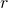,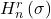and get some identities involving these numbers by using Euler’s transform.

Perfect numbers, Wieferich primes and the solutions of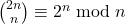Original research paper. Pages 705—712

In this article we focus on the solutions of a congruence equation: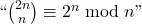. Using the main result of this article and the SageMath software, we improve largely the number of known solutions. Furthermore, we prove that some famous numbers like even perfect numbers and Wieferich primes are connected to solutions of this equation.

Lower bounds on expressions dependent on functions φ(n), ψ(n) and σ(n)
Original research paper. Pages 713—716
Stoyan Dimitrov

The inequalities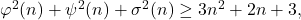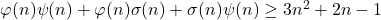connecting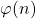,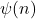and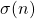-functions are formulated and proved.

Note on the general monic quartic equation
Original research paper. Pages 717—723
Ioana Petkova

In this paper we present a new aproach for solving the general monic quartic equation. Moreover, we show that each quartic equation could be considered as a quasi-reciprocal equation, after a suitable translation of the variable.

Binary expansions of prime reciprocals
Original research paper. Pages 724—736
Brenda Navarro-Flores, José M. González-Barrios and Raúl Rueda

Prime numbers have been always of great interest. In this work, we explore the prime numbers from a sieve other than the Eratosthenes sieve. Given a prime number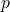, we consider the binary expansion of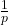and, in particular, the size of the period of. We show some results that relate the size of the period to properties of the prime numbers.

The 2-adic valuation of the general degree-2 polynomial in 2 variables
Original research paper. Pages 737—751
Shubham

We define the-adic valuation tree of a polynomial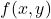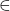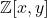by which we can find its-adic valuation at any point. This work includes diverse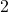-adic valuation trees of certain degree-two polynomials in two variables. Among these, the-adic valuation tree of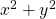is most interesting. We use the observations from these trees to study the-adic valuation tree of the general degree-two polynomial invariables. We also study the-adic valuation tree of the polynomial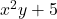.

Notes on generalized and extended Leonardo numbers
Original research paper. Pages 752—773
Anthony G. Shannon, Peter J.-S. Shiue and Shen C. Huang

This paper both extends and generalizes recently published properties which have been developed by many authors for elements of the Leonardo sequence in the context of second-order recursive sequences. It does this by considering the difference equation properties of the homogeneous Fibonacci sequence and the non-homogeneous properties of their Leonardo sequence counterparts. This produces a number of new identities associated with a generalized Leonardo sequence and its associated algorithm, as well as some combinatorial results which lead into elegant properties of hyper-Fibonacci numbers in contrast to their ordinary Fibonacci number analogues, and as a convolution of Fibonacci and Leonardo numbers.

n-Rooks and n-queens problem on planar and modular chessboards with hexagonal cells
Original research paper. Pages 774—788
Eduard C. Taganap and Rainier D. Almuete
We show the existence of solutions to the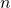-rooks problem and-queens problem on chessboards with hexagonal cells, problems equivalent to certain three and six direction riders on ordinary chessboards. Translating the problems into graph theory problems, we determine the independence number (maximum size of independent set) of rooks graph and queens graph. We consider the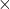planar diamond-shaped Hwith hexagonal cells, and the board Has a flat torus T. Here, a rook can execute moves on lines perpendicular to the six sides of the cell it is placed, and a queen can execute moves on those lines together with lines through the six corners of the cell it is placed.